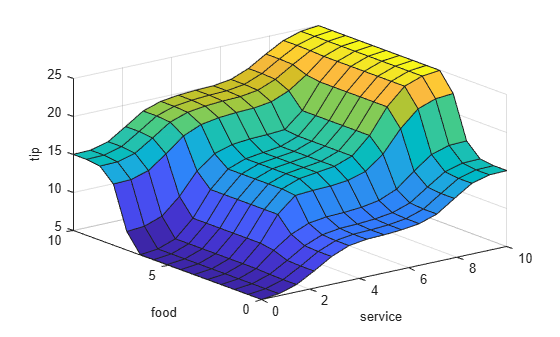# gensurf

Generate fuzzy inference system output surface

## Syntax

``gensurf(fis)``
``gensurf(fis,options)``
``````[X,Y,Z] = gensurf(___)``````

## Description

example

````gensurf(fis)` generates the output surface for the fuzzy inference system, `fis`, plotting the first output variable against the first two input variables. For fuzzy systems with more than two inputs, the remaining input variables use the midpoints of their respective ranges as reference values.```

example

````gensurf(fis,options)` generates the output surface using the specified `options`. To generate a surface using different inputs or outputs, or to specify nondefault plotting options, use this syntax.```

example

``````[X,Y,Z] = gensurf(___)``` returns the variables that define the output surface for any of the previous syntaxes and suppresses the surface plot.```

## Examples

collapse all

Load a fuzzy inference system.

`fis = readfis('tipper');`

This fuzzy system has two inputs and one output.

Generate the output surface for the system.

`gensurf(fis)`Load a fuzzy inference system with two inputs and two outputs.

`fis = readfis('mam22.fis');`

Create a surface generation option set, specifying the second output as the output to plot. By default, this output is plotted against the first two input variables.

`opt = gensurfOptions('OutputIndex',2);`

Plot the surface, using the specified option set.

`gensurf(fis,opt)`Load a fuzzy inference system with four inputs and one output.

`fis = readfis('multiInput.fis');`

Create a default `gensurfOptions` object..

`opt = gensurfOptions;`

Specify plotting options to:

• Plot the output against the second and third input variable.

• Use 20 grid points for both inputs.

• Fix the first and fourth inputs at `-0.5` and `0.1` respectively. Set the reference values for the second and third inputs to `NaN`.

```opt.InputIndex = [2 3]; opt.NumGridPoints = 20; opt.ReferenceInputs = [-0.5 NaN NaN 0.1];```

Plot the output surface.

`gensurf(fis,opt)`Load a fuzzy inference system.

`fis = readfis('tipper');`

Generate the output surface, returning the surface data.

`[X,Y,Z] = gensurf(fis);`

The output values, `Z`, are the FIS output evaluated at the corresponding `X` and `Y` grid points.

## Input Arguments

collapse all

Fuzzy inference system, specified as one of the following:

Surface generation options, specified as a `gensurfOptions` option set.

## Output Arguments

collapse all

Grid values for first input variable, returned as one of the following:

• M-by-N array, where N and M are the number of grid points for the first and second inputs, respectively; that is `options.NumGridPoints = [N M]`. Each column of `X` contains one grid point value, repeated for every row.

• P-element column vector, where P is the number of grid points specified for a single input variable; that is `options.NumGridPoints = P`. Each element of contains one grid point value. This case applies when `fis` has only one input variable.

Grid values for second input variable, returned as one of the following:

• M-by-N array, where N and M are the number of grid points for the first and second inputs respectively; that is `options.NumGridPoints = [N M]`. Each row of `Y` contains one grid point value, repeated for every column.

• `[]` when you specify only one input variable; that is, if you specify `options.InputIndex` as an integer.

Surface output values for the output variable of `fis` specified by `options.OutputIndex`, returned as one of the following:

• M-by-N array, where N and M are the number of grid points for the first and second inputs respectively; that is `options.NumGridPoints = [N M]`. Each element of `Z` is the value of the FIS output, evaluated at the corresponding `X` and `Y` input values. For example, for a two-input system:

`Z(i,j) = evalfis(fis,[X(i,j) Y(i,j)]);`
• P-element column vector, where P is the number of grid points specified for a single input variable; that is `options.NumGridPoints = P`. Each element of `Z` is the value of the FIS output evaluated at the corresponding `X` input value.

When computing the value of `Z`, `gensurf` sets the values of any inputs not specified by `options.InputIndex` to their corresponding reference values, as specified in `options.ReferenceInputs`.

## Alternative Functionality

### App

You can interactively view the control surface for a FIS using the Fuzzy Logic Designer app.

## Version History

Introduced before R2006a

expand all